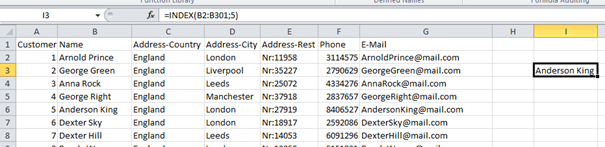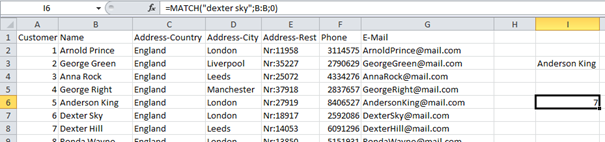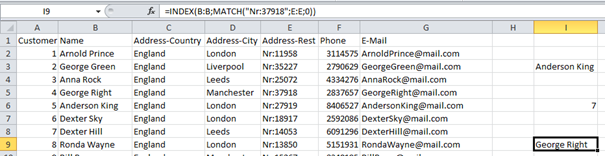# Index-Match Combination

We all love and use Vlookup Function very much. It is a great way to find and fetch values from big lists easily. But Vlookup has a big limitation, it can only search values from left to right. What if the value you are looking for is located on the left side of your lookup value? Index-Match Combination is here for that.

If the value you are looking for is at left side, you need to rearrange whole columns which sometimes contains 10.000’s of rows. Doing this demands a lot of time and patience. But with Index-Match Combination you can fetch values from right to left without moving columns and stuff.

Index Function:

Index function fetches a value from array with a specified position. Syntax for Index function is:

=index(array, index number)

You can inspect the example below. Formula “=INDEX(B2:B301;5)” fetches 5th value from name array, which is “Anderson King”.Match Function:

Match function fetches the position of an item in a list that matches a certain condition. Syntax for match function is:

=match(lookup value; lookup array; match type)

You can inspect the example below. Formula “=MATCH(“dexter sky”;B:B;0)” fetches the position of Dexter Sky in name array, which is “7”.Now that we know how to use both Index Function and Match Function, we can combine them together and make advanced searches. Here is the syntax for Index-Match Combination:

=index(lookup array; match(lookup value; lookup array; match type)

Now you can search any column regardless of its position with index function, all you need is to know index number and it is supplied by match formula. Check below example for better understanding.

We have the door number of a customer and we want to find that customers name. To do this we use this formula “=INDEX(B:B;MATCH(“Nr:37918”;E:E;0))”.  In this formula, Match Function is simply supplying index number for Index Function (which is “4”). So, as seen below, this formula fetches “Name” field (George Right) by using “Address-Rest” field (Nr:37918).